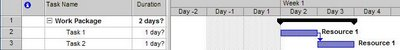# Introduction to EVM – The Toppings## 14 Aug Introduction to EVM – The Toppings

Until now, we have been talking about the key values related to a task. But what about work packages? Let us assume the following example:Since no resources are assigned to the Work Package, we can’t calculate AC, EV and PV directly. You can think of it this way, since the work performed on a work package is the same as the sum of all the work performed so far on the leaf tasks, AC, EV and PV is the sum of all the AC, EV and PV of the tasks that are contained by the work package.

In the case of CV and SV we can either calculate it by using the rollup sum of AC, EV and PV or we can rollup sum CV and SV of the leaf tasks of the work package.

For CPI and SPI we have to calculate it by using AC, EV and PV of the work package. We cannot rollup sum the task indexes.

Until now, we have assumed that the work actually done (EV) is the same as planned. But in some cases it might be different. The way EV is calculated is the planned budget of the task times the physical percent complete. The physical percent complete is an estimation of the resources working on a task and must be given when inserting the work hours in the timesheet.